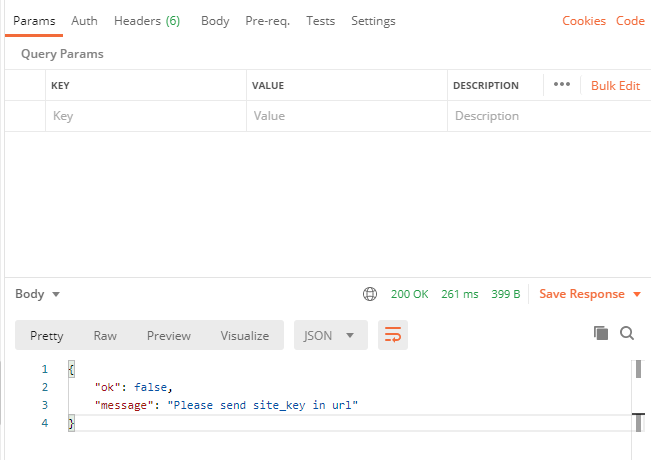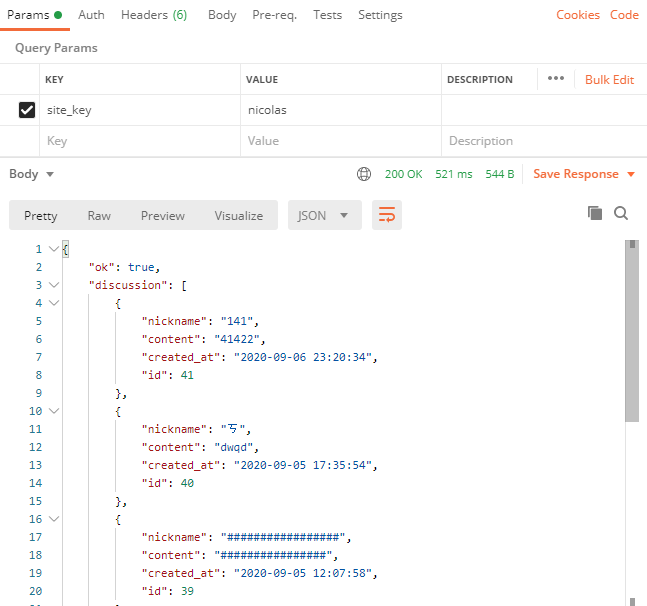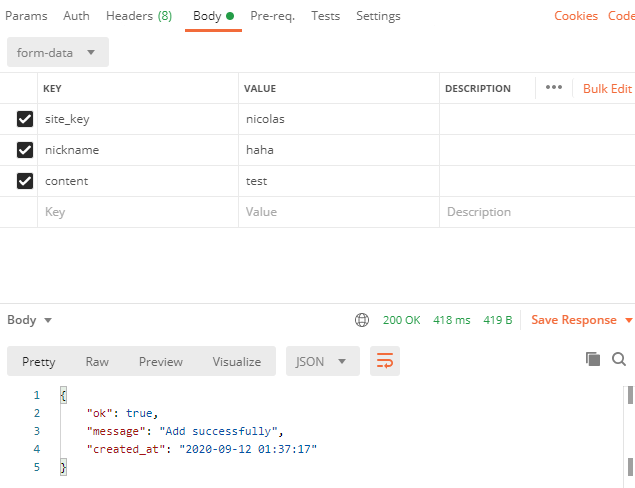# 實作 PHP API & 留言板 SPA（上）

## 開始之前

index.html style.css

script.js

api_comments.php

add_comments_api.php

conn.php

## 後端 PHP 製作 API

api_comments.php

 if (empty($_GET['site_key'])) {$json = [
'ok' => false,
'message' => 'Please send site_key in url'
];
$response = json_encode($json);
echo $response; die(); }  如果有拿到 site_key，我們想把資料庫裡面該 site_key 對應的留言都拿出來並 response 回去，可以這麼寫： 先宣告一個陣列$discusson，然後把每一筆資料都存成一個陣列，再把每一筆資料陣列都 push 進 $discussion 裡。 一樣宣告一個$json 陣列，第一個陣列元素可以放接收成功的訊息 'ok' => true, 第二個陣列元素放我們剛才準備好的 $discussion，最後一樣把這個陣列透過 json_encode($json) 轉換成 JSON 格式，存成 $response 這個變數，最後 echo 出來，代表傳 respsone 出去。 $result = $stmt->get_result();$discussion = [];
while ($row =$result->fetch_assoc()) {
array_push($discussion, [ 'nickname' =>$row['nickname'],
'content' => $row['content'], 'created_at' =>$row['created_at'],
'id' => $row['id'] ]); }$json = [
'ok' => true,
'discussion' => $discussion ];$response = json_encode($json); echo$response;


if(!$result) {$json = [
'ok' => false,
'message' => $conn->error ];$response = json_encode($json); echo$response;
die();
}


<?php
require_once('conn.php');
header('Content-type:application/json;charset=utf-8');
header('Access-Control-Allow-Origin: *');

if (empty($_GET['site_key'])) {$json = [
'ok' => false,
'message' => 'Please send site_key in url'
];
$response = json_encode($json);
echo $response; die(); }$siteKey = $_GET['site_key'];$sql = "SELECT nickname, content, created_at, id FROM nicolakacha_discussion WHERE site_key =? ORDER BY id DESC";
$stmt =$conn->prepare($sql);$stmt->bind_param('s', $siteKey);$result = $stmt->execute(); if(!$result) {
$json = [ 'ok' => false, 'message' =>$conn->error
];
$response = json_encode($json);
echo $response; die(); }$result = $stmt->get_result();$discussion = [];
while ($row =$result->fetch_assoc()) {
array_push($discussion, [ 'nickname' =>$row['nickname'],
'content' => $row['content'], 'created_at' =>$row['created_at'],
'id' => $row['id'] ]); }$json = [
'ok' => true,
'discussion' => $discussion ];$response = json_encode($json); echo$response;
?>add_comments_api.php

 if (
empty($_POST['site_key']) || empty($_POST['nickname']) ||
empty($_POST['content']) ) {$json = [
'ok' => false,
'message' => 'Please input all fields'
];
$response = json_encode($json);
echo $response; die(); }  如果要求的三個 POST 都有，我們就把資料存進去資料庫，如果存成功，就 response 一個會告訴 client 端成功的訊息，若存失敗，就 response 錯誤的訊息，於是完整的程式碼就長這樣： <?php require_once('conn.php'); header('Content-type:application/json;charset=utf-8'); header('Access-Control-Allow-Origin: *'); if ( empty($_POST['site_key']) ||
empty($_POST['nickname']) || empty($_POST['content'])
) {
$json = [ 'ok' => false, 'message' => 'Please input all fields' ];$response = json_encode($json); echo$response;
die();
}
$siteKey =$_POST['site_key'];
$nickname =$_POST['nickname'];
$content =$_POST['content'];
$created_at = date('Y-m-d h:i:s',time()+28800);$sql = "INSERT INTO nicolakacha_discussion(site_key, nickname, content) VALUES (?, ?, ?)";
$stmt =$conn->prepare($sql);$stmt->bind_param('sss', $siteKey,$nickname, $content);$result = $stmt->execute();$json = $result ? [ 'ok' => true, 'message' => 'Add successfully', 'created_at' =>$created_at]
: ['ok' => false, 'msg' => $conn->error];$response = json_encode($json); echo$response;
?>